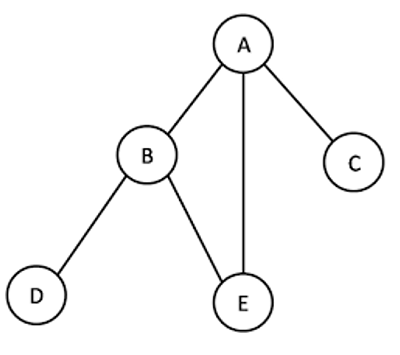# Breadth First Search for a Graph in Python

Python | Breadth First Search: Here, we will learn about Breadth First Search Algorithm and how to implement the BFS algorithm for a graph?
Submitted by Soumya Sinha, on December 30, 2020

A Breadth-first search algorithm is often used for traversing/searching a tree/graph data structure.

Here, we will learn to implement BFS Algorithm for a graph.

BFS for a graph is almost similar to BFS of a tree. There is only one difference here, unlike trees graphs may contain cycles, so it may happen that we come across the same vertex again and again. A vertex needs to be processed only once, so to avoid this situation we will use an array to keep track of the state of the vertex.

For example, in the following graph, suppose we start traversing from vertex A. When we come to vertex B, we look for all adjacent vertices of it. A is also an adjacent vertex of B. If we don't keep track of the visited vertices, then A will be processed again and again, hence this will become a non-terminating process.Description:

In this algorithm we need to discover vertices in order of distance from the source vertex. This breadth first search algorithm works for both directed and undirected graphs.

Data Structures Used:

• state[u]: Provides the colour status of a node during the BFS operation.
• If state[u] = 1, then the node has not been discovered yet.
• If state[u] = 0, then the node has been discovered but not processed yet.
• If state[u] = 0, then the node has been processed.
• distance[u]: Stores the distance of a vertex from the source vertex S
• parent[u]: Stores the parent information

Procedure:

```BFS(G, s)
#Initialize all the vertex except the source vertex
#V[graph] – list of nodes in the graph
for each vertex u ε V [Graph] - {s}
do state[u] = 1
distance[u] = 'inf'
parent[u] = nil
#Initialize the source vertex
state[s] = 0
distance[s] = 0
parent[s] = nil
#Create an empty Queue and an array arr to store the result
queue = []
arr = []
#Insert the source vertex in the queue
Enqueue(queue, s)
#loop until queue is empty
while queue
do u 🡨  Dequeue(queue)
do if state[v] = 1
then state[v] =  0
distance[v] = distance[u]+1
parent[v] =  u
Enqueue(queue, v)
state[u] = -1
```

Time Complexity:

Time Complexity of BFS = O(V+E) where V is number of vertices and E is number of edges.

## Python Code for Breadth First Search for a Graph

```import sys
import math

def bfs(n, edges, s):
#initialize state, distance and parent for all the vertices
state = [0 for i in range(n)]
distance = [float('inf') for i in range(n)]
parent = [-1 for i in range(n)]
#initialize state, distance and parent for the source vertex
state[s] = 1
distance[s] = 0
parent[s] = 'NIL'
queue = []
arr = []
queue.append(s)
#Start discovering the vertices starting from the source vertex
while queue:
x = queue.pop(0)
arr.append(x)
#Start discovering the vertices adjacent to x and store
#information about their parent, distance and state
for i in range(len(edges[x])):
if state[edges[x][i]] == 0:
state[edges[x][i]] = 1
distance[edges[x][i]] = distance[x] + 1
parent[edges[x][i]] = x
queue.append(edges[x][i])
state[x] = -1

return arr

def main():
#input format is described below
n, m, s = map(int, input().split())
edges = {}
for i in range(n):
edges[i] = []
for i in range(m):
a, b = map(int, input().split())
edges[a] += [b]
edges[b] += [a]
for i in range(n):
edges[i].sort()
arr = bfs(n, edges, s)
print(*arr)

if __name__ == '__main__':
main()
```

Input:

Input format:

• First line of input contains the integers n, m s where
• n = number of nodes
• m = number of edges
• s = source node
• Next m lines contain two integers which specifies that the vertices are connected by an edge
```9 13 0
0 1
0 7
1 7
1 2
2 3
2 5
2 8
3 4
3 5
4 5
5 6
6 7
7 8
```

Output:

Output Format: Breadth first traversal starting from source node

```0 1 7 2 6 8 3 5 4
```

Top MCQs

Languages: » C » C++ » C++ STL » Java » Data Structure » C#.Net » Android » Kotlin » SQL
Web Technologies: » PHP » Python » JavaScript » CSS » Ajax » Node.js » Web programming/HTML
Solved programs: » C » C++ » DS » Java » C#
Aptitude que. & ans.: » C » C++ » Java » DBMS
Interview que. & ans.: » C » Embedded C » Java » SEO » HR
CS Subjects: » CS Basics » O.S. » Networks » DBMS » Embedded Systems » Cloud Computing
» Machine learning » CS Organizations » Linux » DOS
More: » Articles » Puzzles » News/Updates